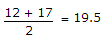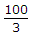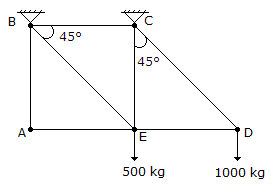# Online Civil Engineering Test - Previous UPSC Exam Papers Test 5Loading Test...

Instruction:

• This is a FREE online test. DO NOT pay money to anyone to attend this test.
• Total number of questions : 20.
• Time alloted : 30 minutes.
• Each question carry 1 mark, no negative marks.
• DO NOT refresh the page.
• All the best :-).

1.

Mercury (density = 13600 kg/m3, σ = 0.49N/mm internal diameter open-ended capillary tube is inserted in the middle of the beaker into the mercury. The meniscus in the tube will be below the external mercury surface by how much distance?

A.
 4.2 mmB.
 5.7 mmC.
 6.8 mmD.
 7.3 mm2.

The length, coefficient of thermal expansion and Young's modulus of bar 'A' are twice that of bar 'B'. If the temperature of both bars is increased by the same amount while preventing any expansion, then the ratio of stress developed in bar A to that in bar B will be

A.
 2B.
 4C.
 8D.
 163.

The compacting factor test of cement concrete determines its

A.
 strengthB.
 porosityC.
 degree of compaction under loadsD.
 workability4.

Self-purification of running streams may be due to

A.
 sedimentation, oxidation land coagulationB.
 dilution, sedimentation and oxidationC.
 dilution, sedimentation and coagulationD.
 dilution, oxidation and coagulation5.

What is the steepest gradient permissible on a 2° curve for B.G. line having using gradient of 1 in 200 ?

A.
 1 in 250B.
 1 in 238C.
 1 in 209D.
 1 in 1986.

Consider the following statements :
A : The collapse load found in the plastic analysis of a continuous beam is affected by sinking of supports.
B : Large elastic stresses are caused at the supports due to sinking of supports.
Of these statements :

A.
 Both A and B are trueB.
 A is true but B is falseC.
 Both A and B are falseD.
 A is false but B is true7.

Which one of the following methods of O-D traffic surveys is conducted for comprehensive analysis of traffic and transportation data ?

A.
 Home interviewB.
 Roadside interviewC.
 Registration number methodD.
 Postcard method8.

Allowable disposable rate of application of sludge on land is determined by

A.
 carbon content of sludgeB.
 nitrogen content of sludgeC.
 phosphorus content of sludgeD.
 potassium content of sludge9.

Why is a proportional flow weir provided in a grit chamber ?

A.
 To reduce the suspended solids entering into the grit chamberB.
 To maintain the constant flow depth in the grit chamberC.
 To take care of maintaining constant flow velocity in the grit chamber over a certain depth rangeD.
 To allow the sewage afresh into the grit chamber10.

Two small orifices A and B of diameters 1 cm and 2 cm, respectively, are placed on the sides of a tank at depths of h1 and h2 below the open liquid surface. If the discharges through A and B are equal, then the ratio of h1 and h2 (assuming equal Cd values) will be

A.
 16 : 1B.
 8 : 1C.
 4 : 1D.
 2 : 111.

Consider the following factors :
1. Reaction time
2. Speed
3. Coefficient of longitudinal friction
Which of these factors are taken into account for computing braking distance ?

A.
 1 and 3B.
 1, 2 and 4C.
 2, 3 and 4D.
 2 and 312.

A 4-hour rainfall in a catchment of 250 km2 produces rainfall depths of 6.2 cm and 5.0 cm in successive 2-hour unit periods. Assuming the φ index of the soil to be 1.2 cm/hour, the run-off volume in ha-m will be

A.
 16B.
 22C.
 1600D.
 220013.

Which of the following are the requirements for the design of a transition curve for a highway system ?
1. Rate of change of grade
2. Rate of change of radial acceleration
3. Rate of change of super elevation
4. Rate of change of curvature
Select the correct answer using the code given below :

A.
 1, 2 and 3B.
 2, 1 and 4C.
 1, 3 and 4D.
 2, 3 and 414.

A star is observed at its upper culmination when it is north of zenith. The latitude of the place of observation is 30°N and declination of the star is 50°N. The zenith distance of the star is

A.
 10°B.
 20°C.
 40°D.
 80°15.

What is the value of camber rate that should be provided in case of WBM pavement surface in an area of heavy rainfall ?

A.
 1 in 30B.
 1 in 48C.
 1 in 60D.
 1 in 7216.

What are leaping weirs ?

A.
 sewage flow measuring devicesB.
 storm regulatorsC.
 velocity control devicesD.
 sewer out falls17.

The area of a plot is to be determined using Simpson's rule. The following offsets were taken to a boundary from points along a chain line all measurements being in metres 12, 15, 22, 29, 36, 38, 31, 22, 17.There were taken at 100 m intervals.
Consider the following steps in this regard.
1.2. Sum of the odd ordinates = 22 + 36 + 1 = 89
3. Sum of the even ordinates = 15 + 29 + 38 + 22 = 104
4. 12 + 17 = 39
5. Area = 100 (19.5 + 89 + 104)
6. Area =(39 + 2 x 89 + 4 x 104)
7. Area 100 x 19.5 (104 - 89)
The area of the plot can be determined by using the steps listed above :

A.
 1, 2, 3 and 5B.
 1, 3, 4 and 6C.
 1, 2, 4 and 7D.
 2, 3, 4 and 618.

The cantilever frame shown in the given figure is supported by vertical links at B and C and carries loads as shown. The force in the bar AE isA.
 500 kgB.
 1000 kgC.
 zeroD.
 2500 kg19.

Which of the following pairs regarding the defects in timber are correctly matched ?
1. Upsets....due to overmaturity and unventilated star age of wood.
2. Fwciness....due to crushing of fibres running transversel.
3. Star shakes....radial splits widest at the circumference and diminishing towards the centre.
4. Heart shakes....cracks widest at the centre and diminishing towards the outer circumference.
Select the correct answer using the codes given below.

A.
 1 and 2B.
 3 and 4C.
 1 and 4D.
 2 and 420.

A 600 mm long and 50 mm diameter rod of steel (E = 200 GPa, α = 12 x 10-6/°C) is attached at the ends to unyielding supports. When the temperature is 30°C there is no stress in the rod. After the temperature of the rod drops to - 20°C, the axial stress in the rod will be

A.
 24 MPa (compressive)B.
 72 MPa (compressive)C.
 120 MPa (tensile)D.
 144 MPa (compressive)